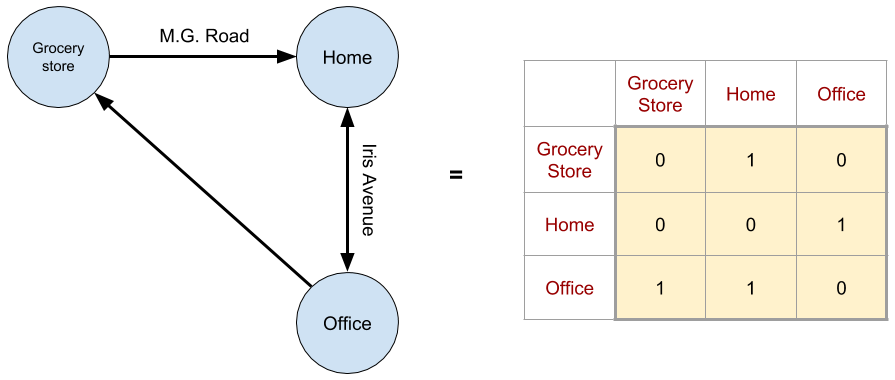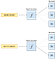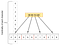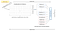# Encoding fixed length high cardinality non-numeric columns for a ML algorithm

ML algorithms work only with numerical values. So there is a need to model a problem and its data completely in numbers. For example, to run a clustering algorithm on a road network, representing the network / graph as an adjacency matrix is one way to model it.Figure 1: An simple road network represented as a Graph, where points of interest are nodes and roads connecting them are edges is shown on the left. The corresponding adjacency matrix is shown on the right

Once transformed to numbers, clustering algorithms like k-means, to identify any underlying structure, can be easily invoked like so

`from sklearn.cluster import KMeansimport numpy as npX = np.array([    [0, 1, 0],     [0, 0, 1],     [1, 1, 0]])kmeans = KMeans(n_clusters=2, random_state=0).fit(X)`

Similarly, a tabular data with a mix of numerical and non-numerical / categorical data also needs to be transformed or encoded to a table of only numbers for a ML algorithm to work on. Columns of string values are quite common in tabular data and in this article, some ideas on how to encode them, especially ones with high cardinality and are of known lengths like IP addresses, mobile numbers etc. are discussed. How would or did you solve this problem? Please comment below. Would like to learn from your experiences as well :)

A traditional way to handle categorical columns is to one-hot encode them. Encoding Browser column in Figure 2, would then become

The Browser column is expanded to 4 columns, which is equal to its cardinality. Cardinality of a column here means the number of unique values in the column and it is 4 in this case — Chrome, Firefox, Safari & Edge.

One-hot encoding a IP address column the same way may result in millions of sparse columns in a realistic situation because in theory, the cardinality of IP addresses is more than 4.29 Billion. Three ways to encode such high cardinality columns are:

1. Bucketing or Hashing
2. Character Encoding
3. Embeddings

# Bucketing or Hashing

This is a commonly used technique to reduce cardinality. Here the idea is to design a function f, which takes a IP Address and returns a number. The catch is, f should only return a fixed set of numbers / buckets. The number of buckets is usually an argument to f. Also every IP Address ever possible should be somehow mapped to one of the fixed number of buckets. As the number of buckets can be chosen to be reasonably small, it can be one-hot encoded like in the case of Browser column.Figure 3: Hashing IPv4 Addresses into fixed number of buckets

The above approach reduces cardinality by capping the number of buckets. But the number of IP Addresses mapped to each bucket can vary a lot if f is not designed with underlying data distribution in mind. Introducing this skew in data due to encoding can impact the performance of the ML algorithm. So, the goal of f is to not only map every IP Address to a bucket, but also to some how maintain the original data distribution as closely as possible.

Another important requirement for f is consistency. Same IP address should be mapped to the same bucket no matter when and how many times it is called. It is easy enough to see why it is required as the ML algorithm would model the data using the encoding alone.

# Character Encodings

Designing an optimal hash function for every categorical column with all constraints as described above can be challenging sometimes. However in the case of IP Address, there is an inherent structure in its digits. Besides the core networking & subnet reasons for its structure, it is also claimed that that it is possible to estimate the geo location from a IP Address. Using these hints f can be designed by one-hot encoding each character. I came up with this simple idea while working on one my projects and it worked really well, which prompted me to write this post as it is something new.Figure 4: Expanding IPv4 Address to fixed 12 characters long by padding each section of it with zero(s). Also showing the cardinality of each character which is at most 10

As shown in figure 4, though the cardinality of IP Address column is huge, the cardinality of each character / digit is only 10 in case of IPv4. Using this fact, each digit can be one-hot encoded resulting in only 10 * 12 = 120 columns instead of millions. This can be extended similarly to IPv6 and can be implemented in few lines of code,

`def transform_ip(ip):    """    If IPv4, equalizes each group and left zero pads to match IPv6 length    If IPv6, converts all to lower case    """    IPV6_LENGTH = 39    IPV4_GROUP_LENGTH = 3 # each group in IPv4 is of this length    if len(ip) < IPV6_LENGTH:        # IPv4 address        groups = ip.split( "." )        equalize_group_length = "".join( map( lambda group: group.zfill(3), groups ))        left_pad_with_zeros = list( equalize_group_length ).zfill( IPV6_LENGTH )        return left_pad_with_zeros    else:        return list(ip.lower())`

`transform_ip` does some pre-processing to an IP Address by equalizing the lengths of IPv4 and IPv6 addresses and zero pads each group in IPv4 to make all 4 groups of length 3.

`from sklearn.preprocessing import CategoricalEncoderdef one_hot_ip(df):    """    Converts the ipAddress column of pandas DataFrame df, to one-hot    Also returns the encoder used    """    enc = CategoricalEncoder()    ip_df = df.ipAddress.apply( lambda ip: transform_ip(ip) ).apply( pd.Series ) # creates separate columns for each char in IP    X_ip = enc.fit_transform( ip_df ).toarray()    return X_ip, enc`

Using `scikit-learn` ‘s `CategoricalEncoder` class, each character / digit is encoded into one of the 10 classes (in case of just IPv4). As of today, `CategoricalEncoder` is yet to be released and can be directly installed from their source code,

`pip install git+git://github.com/scikit-learn/scikit-learn.git`

Encoding this way satisfies all the 3 properties of an ideal f — maintain data distribution, consistent, encode all possible IP Addresses. Similar technique can be applied to any high cardinality non-numeric fixed length columns. However, if `the length of these strings x cardinality` is still huge, Embedding technique is an option.

# Embeddings

Encoding each character can blow up the number of dimensions as described above. Also it may not always convey the hidden relationships among entries in a column. It could be nice if the encoding for New York is closer (via some distance metric) to Boston than San Francisco in a database of restaurants and their locations, because New York is closer to Boston than San Francisco. Geographical distance is just one aspect in the restaurants database case. There can be patterns w.r.t location, life style, types of food and more. Hand designing a function which can encode cities capturing these latent features based on the dataset can be quite challenging.

As contextual relationships among categorical variables (like New York & Boston) change based on the dataset, their encodings / embeddings are trained in a supervised learning problem along with other model parameters. Doing so each categorical variable’s representation / encoding is learned in-context of the problem.

To understand better, say the problem is to predict the Duration column given IP Address and Browser columns as in Figure 2. And to keep it simple, only IP Addresses are encoded using Embeddings, while Browsers are simply one-hot encoded like above.Figure 5: Neural Network architecture showing the training process of Embeddings for IP Addresses. Click here to see a bigger picture.

As shown in Figure 5, an Embedding matrix of shape `(Cardinality of IP Address column, # Latent Features)` is initialized randomly and is trained by an optimization algorithm like Gradient Descent using a cost function, just like any other model parameter, in a supervised learning setting. Latent features are numerical values representing various characteristics of a categorical variable. Here, the neural net was asked to find 12 characteristics (not necessarily understood by us) of IP Address, but it can be any number. Along with one-hot encoded browser features they are fed as inputs to a Deep Neural Network. This way more accurate contextual representations of IP Addresses can be learned (instead of hand coded) which are also more compact than one-hot or character based encoding techniques.

Check out the fast.ai blog post on Embeddings which goes into more details with examples on how these embeddings work, in case the above explanation is not clear.

# Conclusion

Three different ways of encoding non-numerical values were shown.

• One-hot encoding being the simplest of all, works well if the cardinality is small and produces very sparse matrixes.
• Bucketing solves this problem by reducing the cardinality but may introduce unwanted data skews if not careful.
• Character encoding solves the cardinality problem by using these facts of input strings - low cardinality for each character and the fixed length nature of inputs. To some extent domain knowledge is needed to ensure if such an encoding is applicable.
• Embeddings offload the responsibility of encoding completely to the neural network. Higher quality and compact latent features are obtained for free. However that needs the encoding problem to be modeled like a supervised problem and may require a lot of data as there are lot more parameters or knobs for the neural network to tune.

Therefore, based on the use case, one can pick the right strategy to encode categorical data and reap the massive benefits of Machine Learning!

What do you think of these ideas? How did you address similar situations of encoding categorical data for a ML algorithm? Share your experiences and thoughts in the comments :)

Applied Deep Learning Engineer | LinkedIn

## More from Nitin Pasumarthy

Applied Deep Learning Engineer | LinkedIn